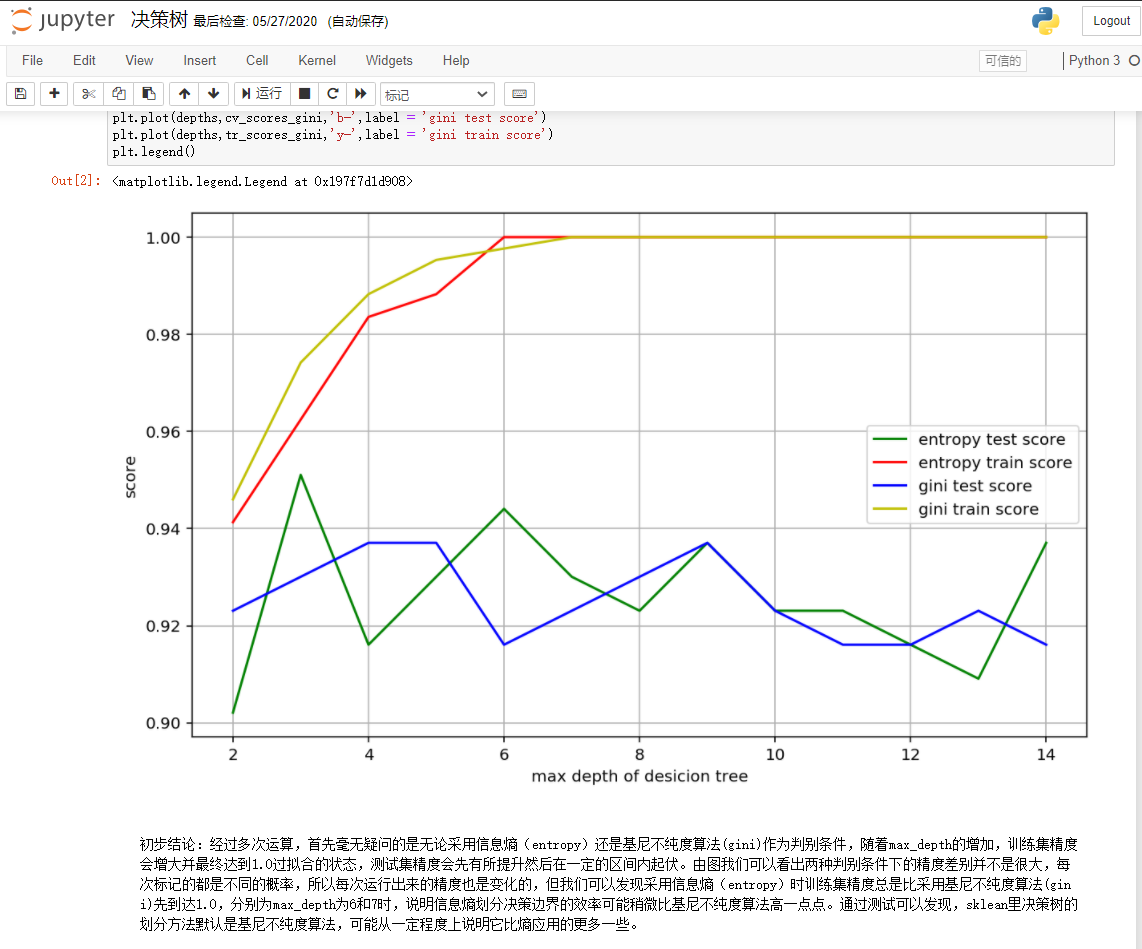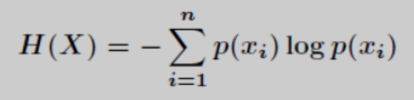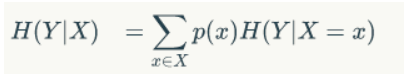# Information entropy and conditional entropy

Time：2022-1-2

## introduction

Today, I suddenly saw the term “information entropy” when I was browsing the paper. I remembered it with a snap. Soon!! Isn’t this the first professional term I talked about in the first introduction to information resource management in my freshman year? I’m familiar with information entropy. I’ll come soon. Information entropy is negative entropy Gan, what is negative entropy. Good guy, the knowledge of a whole course has been returned to the teacher. I only remember the “Jin Ping Mei” recommended by the teacher to us Orz, I’m sorry, teacher. I’m wrong. Run away!!

In order to make atonement, I immediately and soon reviewed the information entropy and the conditional entropy. It’s really not poked!
Okay, just kidding. Because some time ago, when reading a paper on new word discovery, I noticed that the algorithm model takes information entropy as a feature vector as input for mining. Did you find that the original information entropy is also applied in the field of text mining? When you think about it carefully, it seems quite normal that natural language and information theory intersect. Later, baidu looked at it. Good guy, it was also widely used in machine learning. I suddenly remembered that when learning the decision tree model parameter selection algorithm, there were the concepts of entropy and Gini coefficient. It turned out that I still listened carefully, okay? OK, in fact, I only knew the corresponding ID3 and cart algorithms at that time. As for the principle? (forget it, it’s too brain burning. Wouldn’t it be good to be a tuning reference Xia? Which one has high precision and which tube is used so much? Why… Don’t learn from me! Just look at the tears in my eyes and you’ll know ~)
Turn out the assignment at that time??? Good guy, the conclusion written 5 minutes before the deadline of this wave of DDL is very strong. Sure enough, DDL can make us have the courage to give the ship to the teacher. It’s the truth! (I have tested it in practice and it is still in practice)Back to the point, the principle still needs to be understood. In case you can boast one day!!!

## Information entropy

When it comes to information entropy, first of all, what is the amount of information?
As the name suggests, the amount of information can be understood asThe amount of information is a measure of information, just as we can measure time in seconds and love in life Palm your mouth, don’t do business all day (what do you think)
The amount of information is related to the probability of the event. The greater the probability of occurrence, the smaller the amount of information. On the contrary, the smaller the probability of occurrence, the greater the amount of information.In fact, it’s easy to understand. We say that the sun rises in the East. Isn’t that common sense? That’s a small amount of information. But if you say that the sun rises in the west, our first reaction must be??? Indeed, there is a lot of information. If there is not a problem with the sense of orientation, the world will end. Ha ha
of course,The amount of information must not be negative, but it can be 0.(look at what you’re looking at. Don’t make a secret crossing into a warehouse out of nothing. Talk nonsense.)

Therefore, we can roughly summarize as follows:The amount of information of an event is negatively correlated with its occurrence probability, and cannot be negative.

Regardless of the amount of information, let’s start with the formula of information entropy
Suppose P (XI) represents the probability that random event x is Xi, then the information entropy of event x is:In fact, this formula is not complicated. Don’t be confused when you see the formula, okay?
We can clearly see that the information entropy H (x) is related to the logarithm of the event probability p (x).

Back to the amount of information, if there are two unrelated events X and y, we can deduce:
The sum of the amount of information we get from the two events H (x, y) = H (x) + H (y)
Since the two events are not related, the event probability p (x, y) = P (x)P(y)
At this time, it can be preliminarily seen that the logarithms of H (x) and P (x) are related (the real number of multiplication in the logarithm is additive after derivation)
Further derivation, we can get the formula: * * H (x) = – log
2*P(x)**

Is it stupid again? Why is there a minus sign??? Why is the base number 2???
Don’t panic. After reading the big man’s explanation, it suddenly enlightened:

• The minus sign is to ensure that the amount of information remains in a non negative state (after all, you ask for guidance!!)
• The base number is 2: because we only need to meet the formula requirements of low probability events and high amount of information, the choice of logarithm is actually unlimited. But following the general tradition of information theory, we use 2 as the base of logarithm (don’t ask me where the general tradition of information theory came out, I don’t know, hahaha)

Well, the formulas of information entropy and information quantity have been. I believe everyone is obviously aware of their similarities. To sum up:
Entropy is a measure of the amount of information that can be produced before an event
That is: H (x) = sum (P (x) H (x)) = – sum (P (x) log2p (x))
Convert to:## Conditional entropy

What is conditional entropy?
Baidu Encyclopedia tells us: conditional entropy_ H(X|Y)_ Represents a given random variable_ Y_ The uncertainty of random variable x.
Direct up formula:Do you feel the breath of probability theory, as if you know and are not sure? Here is an example:
If there is a black box with 10 balls, 6 white balls and 4 black balls, 7 of them are smooth, of which the white balls are smooth, plus a black ball, and the other 3 black balls are rough, which is obvious:
The probability of touching the white ball is 6 / 10 = 3 / 5
The probability of touching the black ball is: 4 / 10 = 2 / 5
The probability of touching the spherical surface is: 7 / 10 = 7 / 10
The probability of touching the spherical roughness is: 3 / 10 = 3 / 10
We take the color of the touched ball as event y, and we assume that event x is whether the spherical surface of the touched ball is smooth as event X
So at this time
H (y|x = spherical) = P (x = smooth)H (y|x = smooth) + P (x = rough)H (y|x = rough) (1)
It is also known that the information entropy H (y) of event y = – 3 / 5log3 / 5 – 2 / 5log2 / 5 (2)
P (x = smooth) = 7 / 10; (3)
P (x = rough) = 3 / 10; (4)
H (y|x = smooth) = – 6 / 7log6 / 7 – 1 / 7log1 / 7 (6 white balls and 1 black ball out of 7 smooth balls) (5)
H (y|x = rough) = – Log1 (all three rough balls are black balls) (6)

Substitute (2), (3), (4), (5) and (6) into (1) to get the answer!!!
(is there a sense of vision HHH, the teacher said that the formula is complete, but only 2 points will be deducted if the solution is not worked out, and 98 points can be obtained)